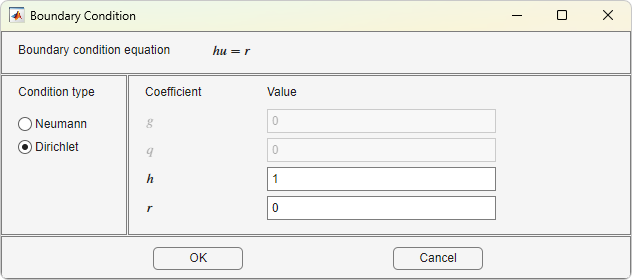Specify Boundary Conditions in the PDE Modeler App

Select Boundary Mode from the Boundary menu or click thebutton. Then select a boundary or multiple boundaries for which you are specifying the conditions. Note that no if you do not select any boundaries, then the specified conditions apply to all boundaries.

• To select a single boundary, click it using the left mouse button.

• To select several boundaries and to deselect them, use Shift+click (or click using the middle mouse button).

• To select all boundaries, use the Select All option from the Edit menu.

Select Specify Boundary Conditions from the Boundary menu.Specify Boundary Conditions opens a dialog box where you can specify the boundary condition for the selected boundary segments. There are three different condition types:

• Generalized Neumann conditions, where the boundary condition is determined by the coefficients q and g according to the following equation:

$\stackrel{\to }{n}\text{\hspace{0.17em}}·\text{\hspace{0.17em}}\left(c\nabla u\right)+qu=g.$

In the system cases, q is a 2-by-2 matrix and g is a 2-by-1 vector.

• Dirichlet conditions: u is specified on the boundary. The boundary condition equation is hu = r, where h is a weight factor that can be applied (normally 1).

In the system cases, h is a 2-by-2 matrix and r is a 2-by-1 vector.

• Mixed boundary conditions (system cases only), which is a mix of Dirichlet and Neumann conditions. q is a 2-by-2 matrix, g is a 2-by-1 vector, h is a 1-by-2 vector, and r is a scalar.

The following figure shows the dialog box for the generic system PDE (Options > Application > Generic System).For boundary condition entries you can use the following variables in a valid MATLAB® expression:

• The 2-D coordinates x and y.

• A boundary segment parameter s, proportional to arc length. s is 0 at the start of the boundary segment and increases to 1 along the boundary segment in the direction indicated by the arrow.

• The outward normal vector components nx and ny. If you need the tangential vector, it can be expressed using nx and ny since tx = –ny and ty = nx.

• The solution u.

• The time t.

Note

If the boundary condition is a function of the solution u, you must use the nonlinear solver. If the boundary condition is a function of the time t, you must choose a parabolic or hyperbolic PDE.

Examples: (100-80*s).*nx, and cos(x.^2)

In the nongeneric application modes, the Description column contains descriptions of the physical interpretation of the boundary condition parameters.

Partial Differential Equation Toolbox DocumentationGet trial now